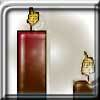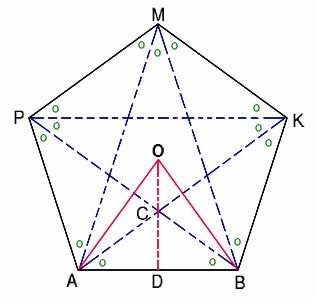#### You may also like### Contact

A circular plate rolls in contact with the sides of a rectangular tray. How much of its circumference comes into contact with the sides of the tray when it rolls around one circuit?Four jewellers share their stock. Can you work out the relative values of their gems?### Burning Down

One night two candles were lit. Can you work out how long each candle was originally?

# At a Glance

##### Age 14 to 16 Challenge Level:Congratulations to all the following who sent in very good solutions to this problem: David from Trinity School, Carlisle (whose solution was the first to arrive); Babak Shirazi from Woodhouse SF College, London; Chen Yiwen from The Chinese High School, Singapore; Nathan from Riccarton High School, Churchtown, New Zealand; Lee Jia Hui from National Junior College, Singapore and finally Alexander from Shevah-Mofet School, Israel. The following solution is made up of bits supplied by several of these contributors.

The pentagon is made of 5 triangles exactly the same as $AOB$, and the pentangle is made of 5 shapes exactly the same as $AOBC$.
\begin{eqnarray} \\ \frac{{\rm Area (pentangle)}}{{\rm Area (pentagon)}} &=& \frac{{\rm Area (AOBC)}}{{\rm Area (AOB)}}\\ &=& 1-\frac{{\rm Area (ACB)}}{{\rm Area( AOB)}} \\ &=& 1-\frac{{\rm Area (DCB)}}{{\rm Area (DOB)}} \\ &=& 1-\frac{DC}{DO}\\ &=& \frac{CO}{DO} < \frac{1}{2}. \end{eqnarray}
In order to calculate this ratio exactly we first find the angles and then use trigonometry. $$\angle AOB = 72^\circ$$ $$\angle DOB = \frac{1}{2}\angle AOB = 36 ^\circ$$As $AC$ is parallel to $PM$ and $CB$ is parallel to $MK$, $$\angle ACB = \angle PMK = 108 ^\circ .$$ $$\angle DCB = {1\over 2}\angle ACB = 54^\circ .$$
\begin{eqnarray} \\ \frac{{\rm Area (pentangle)}}{{\rm Area (pentagon)}} &=& 1-\frac{DB \tan 36}{DB\tan 54}\\ &=& 1-{\tan 36 \over \tan 54} \\ &=& 1- \tan^2 36 \\ &=& 1- (5 - 2{\sqrt 5}) \\ &=& 2{\sqrt 5} - 4 \\ &=& 0.47\quad {\rm approx.} \end{eqnarray}
This ratio is just less than 0.5 meaning the pentangle is a bit smaller than half the pentagon.

Footnote: You don't need a calculator, from the diagram it is possible to calculate exact values for the trig. ratios for $18^\circ$, $36^\circ$, $54^\circ$ and $72^\circ$. All the angles marked with a spot can be shown to be $36^\circ$ using simple properties of triangles. Let $CA=CB=x$. The triangle $PAC$ is an isosceles triangle with base angles of $72^\circ$. If $PA=PC=1$ then $PB=1+x$. Triangles $PAB$ and $ACB$ are similar, hence $$\frac{x}{1} = \frac{1}{1+x}$$

This gives a quadratic equation which can be solved to give $x = \frac{1}{2}({\sqrt 5} - 1).$

Now we have $AD={1\over 2}$ and so (using Pythagoras Theorem to find $CD$): $$\tan^2 36^\circ = 4CD^2 = 4(x^2 - \frac{1}{4}) = 4x^2 - 1 = 5 - 2{\sqrt 5}.$$